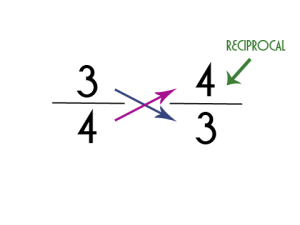# Reciprocal and Division of Fractions

Reciprocal and division of fractions are two different methods. When the numerator and denominator of a fraction are interchanged, then it is said to be its reciprocal. Suppose a fraction is a/b, then its reciprocal will be b/a. A fraction is a numerical quantity that is not a whole number. Rather it represents a part of the whole. For example, it tells how many slices of a pizza are remaining or eating of the whole pizza, such as one-half (½), three-quarters (¾) etc. Division of fractions is an operation performed on fractions with multiple steps. Also, learn the process of dividing fractions here.

Before going forward, let’s have a brief introduction about the parts of fractions and types of fractions. This will help us to find the exact reciprocal of a given fraction as well as in performing the division of fractions.

Parts of Fraction
The fraction has two parts:

Types of Fraction: Fractions are basically of three types, proper, improper and mixed. Learn the definitions below.

Proper Fraction: If both the numerator and denominator are positive, and the numerator is less than the denominator, then it is a proper fraction.

Example: 2/5, 1/3, 3/6, 7/8. 9/11, etc.

Improper Fractions: Fractions having numerators greater than the denominator are called Improper fractions.

Example: 8/3, 3/2, 6/3, 11/9, etc.

Mixed Fraction: When a whole number and a proper fraction are combined, it is known as a mixed fraction.

All these details were the basics of fractions. Now let us learn the reciprocal of fractions along with their division.

## Reciprocal of FractionsThe fraction obtained by swapping or interchanging Numerator and Denominator with each other is known as the Reciprocal of the given fraction.

For example, a reciprocal of 5 is 1/5, a reciprocal of 8/3 is 3/8.

The reciprocal of a mixed fraction can be obtained by converting it into an improper fraction and then swapping the numerator and denominator.

For example, to find the reciprocal of

$$\begin{array}{l}\small 2\frac{1}{3}\end{array}$$
;

• Convert the mixed fraction into improper fraction:
$$\begin{array}{l}\small 2\frac{1}{3}=\frac{7}{3}\end{array}$$
• Now invert the fraction: 7/3 and 3/7, where 3/7 is called reciprocal of 7/3 or
$$\begin{array}{l}\small 2\frac{1}{3}\end{array}$$
.

Note: The product of a fraction and its reciprocal is always 1.

## Division of Fractions

Division involving a fraction follows certain rules. To perform any division involving a fraction just multiply the first number with the reciprocal of the second number. The steps are as follows:

Step 1: First change the division sign (÷) to the multiplication sign (×)

Step 2: If we change the sign of division to multiplication, at the same time we have to write the reciprocal of the second term or fraction.

Step 3: Now, multiply the numbers and simplify the result.

These rules are common for:

1. Division of the whole number by a fraction.
2. Division of a fraction by a whole number
3. Division of a fraction by another fraction.

Note: It is to be noted that division of fractions is basically the multiplication of fractions obtained by reciprocal of the denominator (i.e. divisor).

## Examples of Divisions of Fractions

Examples for each of the conditions mentioned earlier are explained below. These solved examples help you to understand the division of fractions in a better way.

### Division of the Whole Number by a Fraction

Example 1: 16 ÷ 4/3

Solution: 16 ÷ 4/3 = 16/1 × 3/4

3/4 is the reciprocal of 4/3.

Hence, (16 × 3)/(1×4)

× 3 = 12

Therefore,

16 ÷ 4/3 = 12

### Division of a Fraction by a Whole Number

Example 2: Divide 8/3 by 3

Solution: We need to simplify, 8/3 ÷ 3

The reciprocal of 3 is 1/3.

Now writing the given expression into multiplication form,

8/3 × 1/3 = 8 /9

Therefore,

8/3 ÷ 3 = 8/9

### Division of a Fraction by another Fraction

Example 3: 8/3 ÷ 4/3

Solution: 8/3 ÷ 4/3

The reciprocal of second term 4/3 is 3/4.

Now multiply the first term with the reciprocal of the second term.

8/3 × 3/4 = 8/4 = 2

Hence,

8/3 ÷ 4/3 = 2

To perform division involving mixed fraction, convert the mixed fraction into an improper fraction and follow the above steps.

## Video Lesson on Fractions### Practice Problems

1. Divide 25/4 by 16.
2. What is the reciprocal of -9/7?
3. Perform the division of fractions 7/48 and 32/9.

Study more on the related topics such as representing fractions on a number line at BYJU’S today!

## Frequently Asked Questions – FAQs

Q1

### What is a reciprocal of the fraction?

The reciprocal of a fraction will obtain by interchanging the numerator and denominator. For example, y/x is the reciprocal of the fraction x/y, i.e. y/x = 1/(x/y).
Q2

### How do you divide fractions with whole numbers?

When dividing fractions by whole numbers, we should convert the division into multiplication by writing the reciprocal of the divisor, i.e. a whole number. For example, dividing 2/3 by 2 can be performed by converting as (2/3) × (1/2). Hence, simplification becomes easy now.
Q3

### Why do you use reciprocal when dividing fractions?

To simplify the division process when dividing fractions, reciprocals are used so that division will be converted to multiplication. For example, (4/5) ÷ (8/7) can be written as (4/5) × (7/8).
Q4

### What is the reciprocal rule of division?

The reciprocal rule of division method is “Multiply the dividend by the reciprocal of the divisor”. In simple words, invert the divisor and multiply with the dividend.
Q5

### Is 1 the reciprocal of 1?

Yes, 1 is the reciprocal of 1 itself. Since 1 can be written as 1/1 (i.e. 1/1 = 1 and 1 × 1 = 1).
Q6

### What are the different cases involved in the division of fractions?

There are three different cases that exist in the division of fractions such as:
Dividing a fraction by a whole number
Dividing a whole number by a fraction
Dividing a fraction by another fraction (i.e. division of two fractions)
Test your Knowledge on Reciprocal and Division of Fractions